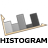You are here: Start » AVL.NET » Histogram Combinators

# Histogram Combinators

IconNameDescriptionAddHistogramsAdds two histograms bar by bar.AddHistograms_OfArrayAdds histograms of an array bar by bar.AddHistograms_OfLoopAdds histograms appearing in consecutive iterations bar by bar.DifferenceHistogramComputes histogram representing difference between corresponding bars of given histograms.DivideHistogramsDivides two histograms bar by bar.MaximumHistogramComputes the maximum of two histograms bar by bar.MaximumHistogram_OfArrayComputes the maximum of histograms of an array bar by bar.MaximumHistogram_OfLoopComputes the maximum of histograms appearing in consecutive iterations bar by bar.MinimumHistogramComputes the minimum of two histograms bar by bar.MinimumHistogram_OfArrayComputes the minimum of histograms of an array bar by bar.MinimumHistogram_OfLoopComputes the minimum of histograms appearing in consecutive iterations bar by bar.MultiplyHistogramsMultiplies two histograms bar by bar.MultiplyHistograms_OfArrayMultiply histograms of an array bar by bar.MultiplyHistograms_OfLoopMultiply histograms appearing in consecutive iterations bar by bar.SubtractHistogramsSubtracts two histograms bar by bar.